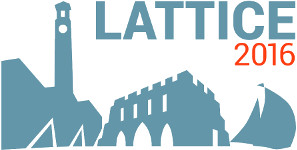#The 34th International Symposium on Lattice Field Theory (Lattice 2016)

Jul 24 – 30, 2016
Highfield Campus, University of Southampton
Europe/London timezone

## Lattice QCD calculation of form factors for $\Lambda_b \to \Lambda(1520) \ell^+ \ell^-$ decays

Jul 29, 2016, 4:50 PM
20m
Building 67 Room 1003 (Highfield Campus, University of Southampton)

### Building 67 Room 1003

#### Highfield Campus, University of Southampton

Highfield Campus, Southampton SO17 1BJ, UK
Talk Weak Decays and Matrix Elements

### Speaker

Prof. Stefan Meinel (University of Arizona / RIKEN BNL Research Center)

### Description

Experimental results for mesonic $b \to s \mu^+ \mu^-$ decays show a pattern of deviations from Standard-Model predictions. These deviations could be due to new fundamental physics or due to an insufficient understanding of hadronic effects. Complementary information on the $b \to s \mu^+ \mu^-$ transition can be obtained from $\Lambda_b$ decays. This was recently done using the process $\Lambda_b \to \Lambda \mu^+ \mu^-$, where the $\Lambda$ is the lightest strange baryon. A further interesting channel that is being analyzed by LHCb is $\Lambda_b \to p^+ K^- \mu^+ \mu^-$, where the $p^+ K^-$ final state receives contributions from multiple higher-mass $\Lambda$ resonances. The narrowest and most prominent of these is the $\Lambda(1520)$, which has $J^P=\frac32^-$. We discuss our progress toward a lattice QCD calculation of the relevant $\Lambda_b \to \Lambda(1520)$ form factors.

### Primary authors

Gumaro Rendon (University of Arizona) Prof. Stefan Meinel (University of Arizona / RIKEN BNL Research Center)

 Slides